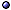Types and Examples of Categories

General Types
* Single spaces as categories: Any set with a relation is a category, with relations as arrows; For example, a poset.
* Thin: One for which each Hom-set contains at most one element; For example, a poset.
* With terminal element: One with an object to which there is an arrow from every other object; For example, Set.
* Tensor categories: Types are spherical, ribbon, symmetric; > s.a. lattice gauge theory.
* Remark: Some categories are modeled after other ones; For example, manifolds are modeled after finite-dimensional vector spaces.
@ Other types: Neeman Top(98) [non-compactly generated]; Jacobs a1101 [dagger categories of tame relations].

Exampless.a. category theory [generalizations]; categories in physics [categories of relations, applications].
* Set: The category of sets and mappings between sets.
* Top: The category of topological spaces, with continuous maps as morphisms; The composition is composition of maps.
* Grp: The category of groups, with homomorphisms as morphisms; Composition is composition of maps; A monomorphism is a 1-1 homomorphism f: Ker(f) = e (the identity).
* Hilb: The category of Hilbert spaces and bounded linear operators, used in quantum mechanics.
* Man: The category of differentiable manifolds and differentiable maps.
* nCob: The category of manifolds (= spacelike hypersurfaces) and n-dimensional cobordisms, used in general relativity.
* Prop: A strict symmetric monoidal category where the objects are natural numbers, with the tensor product of objects given by addition.
* Vec: The category of vector spaces, a monoidal tensor category.
* R-modules: A monomorphism μ: AB is essential if for any submodule H of B, if H ≠ 0 then HμA ≠ 0.
* Non-trivial category: @ see Spanier 66 on algebraic topology; The composition is not the usual composition of maps.
@ Other examples: Mitchener PLMS(02) [C* categories]; Baez et al a1707 [props, and network theory].
> Other examples: see posets [as (0,1)-categories or thin categories]; fiber bundles [natural bundles].